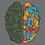# Dangerous sigma

For any positive integer $n$, let $n'$ denote the closest integer to $\sqrt n$, then $\displaystyle \sum_{n=1}^\infty \frac{2^{n'} + \left(\frac12 \right)^{n'}}{2^n} = \ ?$

I don't seem to understand how to even approach this question, any help would be appreciated.

Edit: The question has been turned into a problem by @Chew-Seong Cheong sir. It can be accessed here.1 year, 3 months ago

This discussion board is a place to discuss our Daily Challenges and the math and science related to those challenges. Explanations are more than just a solution — they should explain the steps and thinking strategies that you used to obtain the solution. Comments should further the discussion of math and science.

When posting on Brilliant:

• Use the emojis to react to an explanation, whether you're congratulating a job well done , or just really confused .
• Ask specific questions about the challenge or the steps in somebody's explanation. Well-posed questions can add a lot to the discussion, but posting "I don't understand!" doesn't help anyone.
• Try to contribute something new to the discussion, whether it is an extension, generalization or other idea related to the challenge.

MarkdownAppears as
*italics* or _italics_ italics
**bold** or __bold__ bold
- bulleted- list
• bulleted
• list
1. numbered2. list
1. numbered
2. list
Note: you must add a full line of space before and after lists for them to show up correctly
paragraph 1paragraph 2

paragraph 1

paragraph 2

[example link](https://brilliant.org)example link
> This is a quote
This is a quote
    # I indented these lines
# 4 spaces, and now they show
# up as a code block.

print "hello world"
# I indented these lines
# 4 spaces, and now they show
# up as a code block.

print "hello world"
MathAppears as
Remember to wrap math in $$ ... $$ or $ ... $ to ensure proper formatting.
2 \times 3 $2 \times 3$
2^{34} $2^{34}$
a_{i-1} $a_{i-1}$
\frac{2}{3} $\frac{2}{3}$
\sqrt{2} $\sqrt{2}$
\sum_{i=1}^3 $\sum_{i=1}^3$
\sin \theta $\sin \theta$
\boxed{123} $\boxed{123}$

Sort by:

Using Wolfram Alpha the answer is 3. See here.

- 1 year, 3 months ago

- 1 year, 3 months ago

I would not want to try. No algebraic method to handle nearest integers $\lfloor x + \frac 12 \rfloor$.

- 1 year, 3 months ago

OK. I will try if I got I will set it as a question. I have some idea now.

- 1 year, 3 months ago

Check out the solution here.

- 1 year, 3 months ago

Thank you sir! Very grateful.

- 1 year, 3 months ago

Did you delete the solution to my recent problem? @Chew-Seong Cheong

- 1 year ago

Yes, I don't think my solution was correct.

- 1 year ago